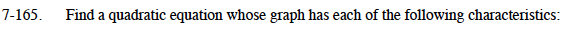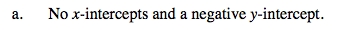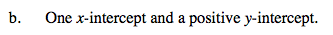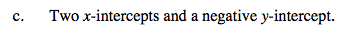Home > CCA2 > Chapter 7 > Lesson 7.2.4 > Problem7-165

7-165.
1. Find a quadratic equation whose graph has each of the following characteristics: 7-165 HW eTool (Desmos). Homework Help ✎

1. No x−intercepts and a negative y-intercept.

2. One x−intercept and a positive y-intercept.

3. Two x-intercepts and a negative y-intercept.Write the equation of an inverted parabola with a vertex below the x-axis.This parabola will sit on the x-axis.Shift the graph of y = x² down.

Use the eTool below to explore the problem.
Click the link at right for the full version of the eTool: CCA2 7-165 HW eTool